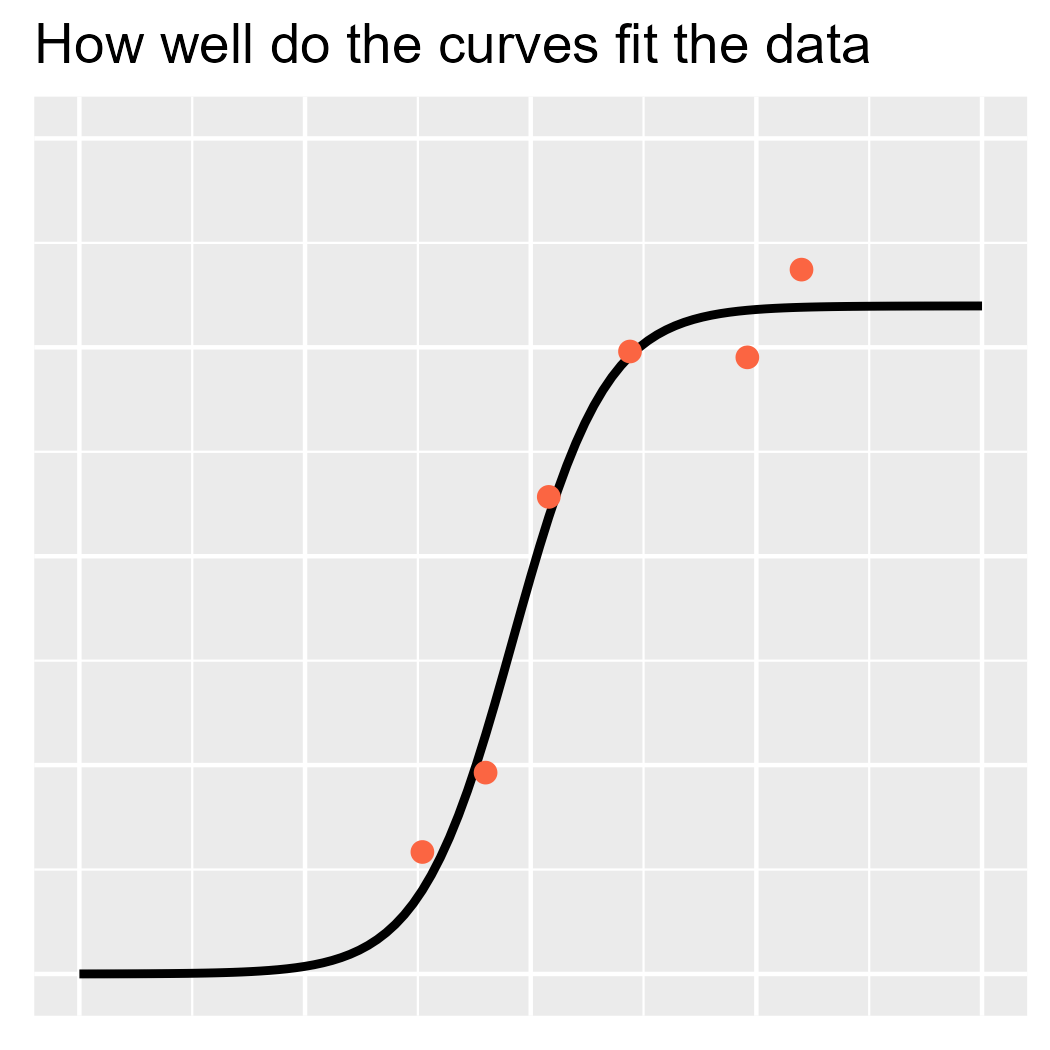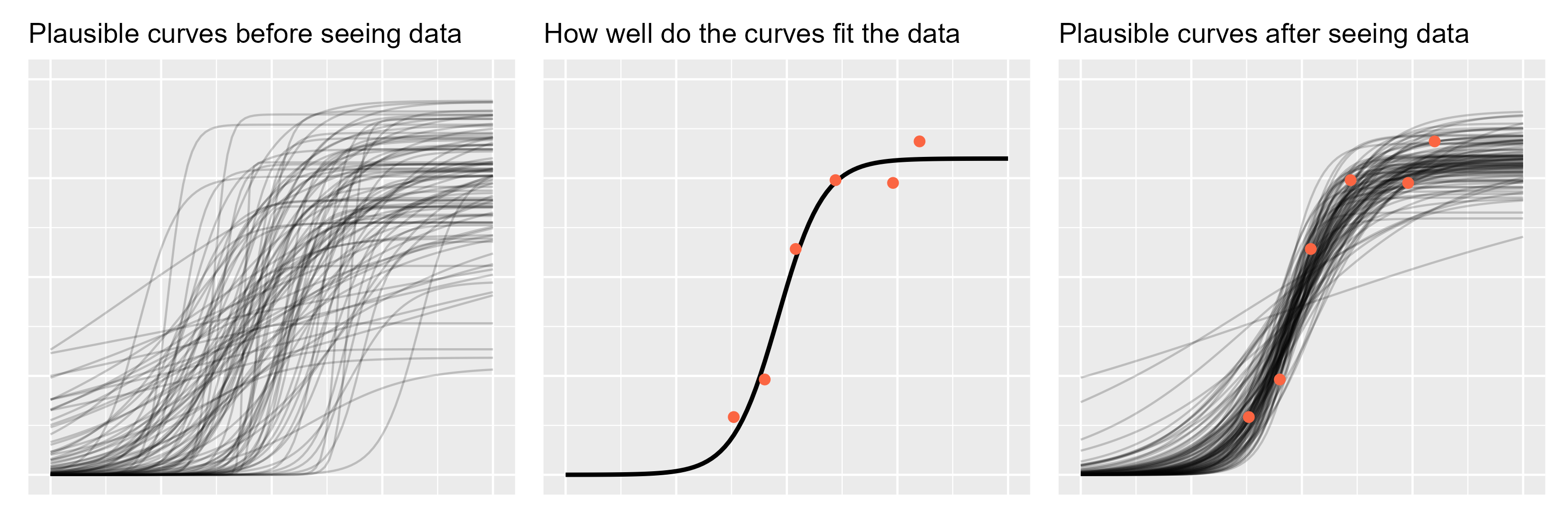Want to share your content on R-bloggers? click here if you have a blog, or here if you don't.

In my last post, I walked through
an intuition-building visualization I created to describe mixed-effects models
for a nonspecialist audience. For that presentation, I also created an analogous
visualization to introduce Bayes’ Theorem, so here I will walk through that
figure..

As in the earlier post, let’s start by looking at the visualization and
then we will recreate it using a simpler model and smaller dataset.

## Names for things

First, let’s review the theorem. Mathematically, it says how to convert one
conditional probability into another one.

The formula becomes more interesting in the context of statistical modeling. We
have some model that describes a data-generating process and we have some
observed data, but we want to estimate some unknown model parameters.
In that case, the formula reads like:

When I was first learning Bayes, this form was my anchor for remember the
formula. The goal is to learn about unknown quantity from data, so the left side
needs be “unknown given data”.

These terms have conventional names:

Prior and posterior describe when information is obtained: what we know pre-data is our
prior information, and what we learn post-data is the updated information
(“posterior”).

The likelihood in the equation says how likely the data is given the model
parameters. I think of it as fit: How well do the parameters fit the data?
Classical regression’s line of best fit is the maximum likelihood line. The
likelihood also encompasses the data-generating process behind the model. For
example, if we assume that the observed data is normally distributed, then we
evaluate the likelihood by using the normal probability density function. You
don’t need to know what that last sentence means. What’s important is that the
likelihood contains our built-in assumptions about how the data is distributed.

The average likelihood—sometimes called evidence—is weird. I don’t have a
script for how to describe it in an intuitive way. It’s there to make sure the
math works out so that the posterior probabilities sum to 1. Some presentations
of Bayes theorem gloss over it, noting that the posterior is proportion to the
likelihood and prior information.

There it is. Update your prior information in proportion to how well it fits
the observed data.
My plot about Bayes’ theorem is really just this form of the
equation expressed visually.For my birthday last year, I got a shirt with Bayes’ theorem on it.

## The model: nonlinear beta regression

The data I presented at the conference involved the same kinds of logistic growth
curves I wrote about last year
. I will
use the same example dataset as in that post.

library(tidyverse)
#> -- Attaching packages --------------------------------------- tidyverse 1.3.0 --
#> √ ggplot2 3.2.1     √ purrr   0.3.3
#> √ tibble  2.1.3     √ stringr 1.4.0
#> √ tidyr   1.0.2     √ forcats 0.5.0
#> Warning: package 'forcats' was built under R version 3.6.3
#> -- Conflicts ------------------------------------------ tidyverse_conflicts() --

data <- tibble(
age = c(38, 45, 52, 61, 80, 74),
prop = c(0.146, 0.241, 0.571, 0.745, 0.843, 0.738)
)


Here x is a child’s age in months and y is
how intelligible the child’s speech is to strangers as a proportion. We use a
nonlinear beta regression model. The beta regression handles the fact that the
data are proportions, and the nonlinear encodes some assumptions about growth:
it starts at 0, reaches some asymptote, etc. Finally, our prior information
comes from our knowledge about when and how children learn to talk. (Nobody is
talking in understandable sentences at 16 months of age.)

Here is the model specification. I won’t go over it in detail.

library(brms)
#> can be found by typing help('brms'). A more detailed introduction
#> to the package is available through vignette('brms_overview').
#>
#> Attaching package: 'brms'
#> The following object is masked from 'package:stats':
#>
#>     ar

inv_logit <- function(x) 1 / (1 + exp(-x))

model_formula <- bf(
# Logistic curve
prop ~ inv_logit(asymlogit) * inv(1 + exp((mid - age) * exp(scale))),
# Each term in the logistic equation gets a linear model
asymlogit ~ 1,
mid ~ 1,
scale ~ 1,
# Precision
phi ~ 1,
# This is a nonlinear Beta regression model
nl = TRUE,
)

prior_fixef <- c(
# Point of steepest growth is age 4 plus/minus 2 years
prior(normal(48, 12), nlpar = "mid", coef = "Intercept"),
prior(normal(1.25, .75), nlpar = "asymlogit", coef = "Intercept"),
prior(normal(-2, 1), nlpar = "scale", coef = "Intercept")
)

prior_phi <- c(
prior(normal(2, 1), dpar = "phi", class = "Intercept")
)


## Sampling from the prior

Bayesian models are generative. They describe a data-generating process, so they
can be used to simulate new observations. Richard McElreath sometimes uses the
language

of running a model forwards to generate new observations using parameters and
running it backwards to infer the data-generating parameters from the data.

If we don’t have any data in hand, then running the model forwards to simulate
data will using only the prior. I am not going as far as simulating actual
observations; rather I will sample regression lines from the prior. These
samples represent growth trajectories that are plausible before seeing any data.

We can use sample_prior = "only" to have brms ignore the data and sample from
the prior distribution.

fit_prior <- brm(
model_formula,
data = data,
prior = c(prior_fixef, prior_phi),
iter = 2000,
chains = 4,
sample_prior = "only",
cores = 1,
control = list(adapt_delta = 0.9, max_treedepth = 15)
)
#> Compiling the C++ model
#> Start sampling


We can randomly draw some lines from the prior distribution by using
add_fitted_draws() from the tidybayes package.

draws_prior <- data %>%
tidyr::expand(age = 0:100) %>%

p1 <- ggplot(draws_prior) +
aes(x = age, y = .value) +
geom_line(aes(group = .draw), alpha = .2) +
theme(
axis.ticks = element_blank(),
axis.text = element_blank(),
axis.title = element_blank()
) +
expand_limits(y = 0:1) +
ggtitle("Plausible curves before seeing data")
p1A word of encouragement! The prior is an intimidating part of Bayesian
statistics. It seems highly subjective, as though we are pulling numbers from
thin air, and it can be overwhelming for complex models. But if we are familiar
with the kind of data we are modeling, we have prior information. We can have
the model simulate new observations using the prior distribution and then
plot the hypothetical data. Does anything look wrong or implausible about the
simulated data? If so, then we have some prior information that we can include
in our model. Note that we do not evaluate the plausibility of the simulated
data based on the data we have in hand (the data we want to model); that’s not
prior information.

## Finding the best fit

To illustrate the likelihood, I am going to visualize a curve with a very high
likelihood. I won’t use Bayes here; instead, I will use nonlinear least squares
nls() to illustrate what a purely data-driven fit would be. This approach is
not the same as finding the best-fitting line from the posterior
distribution—waves hands—but hey, we’re just building intuitions here.

# Maximum likelihood estimate
fm1 <- nls(prop ~ SSlogis(age, Asym, xmid, scal), data)
new_data <- tibble(age = 0:100) %>%
mutate(
fit = predict(fm1, newdata = .)
)

point_orange <- "#FB6542"

p2 <- ggplot(data) +
aes(x = age, y = prop) +
geom_line(aes(y = fit), data = new_data, size = 1) +
geom_point(color = point_orange, size = 2) +
theme(
axis.ticks = element_blank(),
axis.text = element_blank(),
axis.title = element_blank()
) +
expand_limits(y = 0:1) +
expand_limits(x = c(0, 100)) +
ggtitle("How well do the curves fit the data")
p2## Sample from the posterior

Now, let’s fit the actual model and randomly draw regression lines from the
posterior distribution.

fit <- brm(
model_formula,
data = data,
prior = c(prior_fixef, prior_phi),
iter = 2000,
chains = 4,
cores = 1,
control = list(adapt_delta = 0.9, max_treedepth = 15)
)
#> Compiling the C++ model
#> recompiling to avoid crashing R session
#> Start sampling

draws_posterior <- data %>%
tidyr::expand(age = 0:100) %>%


And now let’s plot the curves with the data, as we have data in hand now.

p3 <- ggplot(draws_posterior) +
aes(x = age, y = .value) +
geom_line(aes(group = .draw), alpha = .2) +
geom_point(
aes(y = prop),
color = point_orange, size = 2,
data = data
) +
theme(
axis.ticks = element_blank(),
axis.text = element_blank(),
axis.title = element_blank()
) +
expand_limits(y = 0:1) +
ggtitle("Plausible curves after seeing data")
p3Finally, we can assemble everything into one nice plot.

library(patchwork)
p1 + p2 + p3## A nice echo

3Blue1Brown is a YouTube channel that specializes in visualizing mathematical
concepts. It’s amazing. About a month after my presentation, the channel
covered Bayes’
theorem
. The
approach was more basic: It looked at probability as discrete frequency counts
and using Bayes to synthesize different frequency probabilities together. Think
of the familiar illness-screening puzzle: x% of positive tests are accurate, y%
of the population has the illness, what is the chance of having the illness
given a positive test? And yet, the video recapped Bayes’ theorem with a
three-panel visualization: